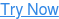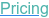#### SOFTWARE#### KNOWLEDGE BASE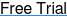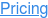# Sample Size for Regression Models

February 7, 2023

In this webinar, we review conventional regression models such as linear regression, logistic regression and Cox regression and examine the use of mixed-effects models in cluster randomized trials.

We investigate sample size determination for these models and contrast their benefits and limitations in different scenarios. We also explore methods for calculating the minimum sample size for clinical prediction models.

## Sample Size for Regression ModelsCalculations for Common Regression, Mixed-Effects and Prediction Models### In this free webinaryou will learn about:

• Regression Models and Sample Size Calculation for Common Regression Models
• Sample Size Determination for Cluster Randomized Designs using Mixed-effects Models
• How to Compute the Minimum Sample Size for Clinical Prediction Models

Regression models are types of statistical models often used in clinical trials to describe the relationship between an outcome variable and one or more predictor variable(s).

Simple linear regression, multiple linear regression, logistic regression and Cox regression are all examples of regression models that can be used in various scenarios. We investigate practical examples showing how to calculate the sample size when these models are used.

Mixed-effects or hierarchical models are another example of regression models typically used in clinical trials, particularly in the analysis of Cluster randomized trials (CRTs). CRTs are a type of experimental design which can be more efficient and cost-effective than individually randomized trials, leading to smaller sample sizes and reduced variance in outcome, however they come with their own challenges and limitations.

We explore the use of Mixed-effects models in these scenarios and provide practical examples of how to determine the sample size for these models.

Minimum sample size requirements for clinical prediction models in the past have often been set using guidelines such as the 10 subjects per predictor variable rule-of-thumb. In recent years many methods have been developed in order to provide a more rigorous approach to determining the minimum sample size for clinical prediction models.

We provide an analysis of the various regression models that can be used for clinical trials and discuss how the sample size can be computed for each. We also discuss the benefits and limitations of each model and investigate methods for computing the minimum sample size for clinical prediction models.

### Play the video below to watchthe complete recording of this webinar

Duration: 60 minutes

 Explore The Data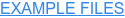Get The Deck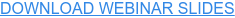• Compute Minimum Sample Size for Linear Prediction Model with Continuous Endpoints Example
• Cox Regression Example
• Linear Regression for One Normal Covariate Example
• Logistic Regression for One Normal Covariate Example
• Mixed-effects Model for 2 Level Hierarchical Design Example

Are you looking for more trial design resources
Webinars to improve clinical trial designs & Practical examples of sample size determination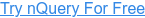## You May Also Like

These Stories on Guide to Sample Size